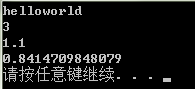# Lua中调用C函数(lua-5.2.3)

Lua可以调用C函数的能力将极大的提高Lua的可扩展性和可用性。

``````// testlua.cpp : 定义控制台应用程序的入口点。
//

#include "stdafx.h"
#include <stdio.h>
#include <string.h>
#include <math.h>

extern "C"
{
#include <lua.h>
#include <lualib.h>
#include <lauxlib.h>
}

//待Lua调用的C注册函数
{
//检查栈中的参数是否合法，1表示Lua调用时的第一个参数(从左到右)，依此类推。
//如果Lua代码在调用时传递的参数不为number，该函数将报错并终止程序的执行。
double op1 = luaL_checknumber(L,1);
double op2 = luaL_checknumber(L,2);
//将函数的结果压入栈中。如果有多个返回值，可以在这里多次压入栈中。
lua_pushnumber(L,op1 + op2);
//返回值用于提示该C函数的返回值数量，即压入栈中的返回值数量。
return 1;
}

//待Lua调用的C注册函数。
static int sub2(lua_State* L)
{
double op1 = luaL_checknumber(L,1);
double op2 = luaL_checknumber(L,2);
lua_pushnumber(L,op1 - op2);
return 1;
}

//待Lua调用的C注册函数。
static int l_sin (lua_State *L) {
double d = lua_tonumber(L, 1); /* get argument */
lua_pushnumber(L, sin(d)); /* push result */
return 1; /* number of results */
}

int _tmain(int argc, _TCHAR* argv[])
{
lua_State *L = luaL_newstate();
luaL_openlibs(L);

//将指定的函数注册为Lua的全局函数变量，其中第一个字符串参数为Lua代码
//在调用C函数时使用的全局函数名，第二个参数为实际C函数的指针。
lua_register(L, "sub2", sub2);
lua_register(L, "l_sin", l_sin);
//在注册完所有的C函数之后，即可在Lua的代码块中使用这些已经注册的C函数了。
luaL_dofile(L,"test.lua");

//if (luaL_dostring(L,testfunc))
// printf("Failed to invoke.\n");

//const char *buf = "print('Hello World')";
//luaL_dostring(L,buf);

lua_close(L);
return 0;
}``````

test.lua

``````function show()
print("helloworld")
print(sub2(20.1,19))
print(l_sin(1))
end

show()  ``````## 您可能还喜欢：

App下载